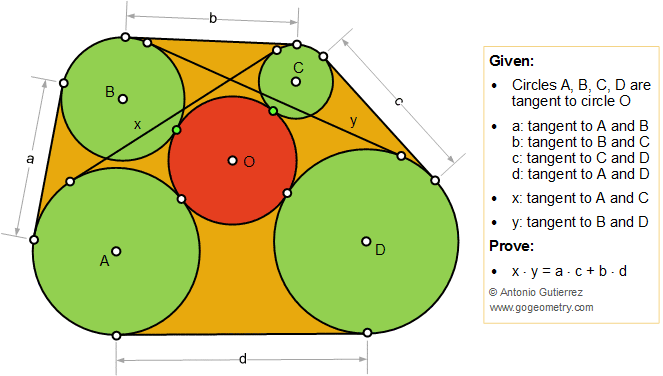# Geometry Problem 1121

## Casey's Theorem. Generalized Ptolemy's Theorem, Art, Poster### Proposition

In the figure below, the circles A,B,C,D are tangent to the circle O; a, b, c, d, x, y are the length of the exterior common tangent to circles A and B, B and C, C and D, D and A, A and C, and B and D respectively. Prove the generalized Ptolemy's theorem: $$x \cdot y = a \cdot c + b\cdot d$$.

#### Figure 1#### Figure 2### Geometric Art using iPad or Mobile Apps

Geometric art is a form of art based on the use and application of geometric figures. A geometric figure is any set or combination of points, lines, surfaces and solids.

A mobile app or mobile application software is a computer program designed to run on smartphones and tablet computers.